Home » LEN Function DAX

# LEN Function DAX### LEN Function is a Text Function that returns the number of characters in a text string. It returns a integer value.

• LEN Function always counts each character as 1.
• Spaces count as characters.
• LEN function implicitly casts the value to text for non- text values such as dates or Boolean.

SYNTAX

`LEN(<text>)`

text is the column or value that contains the text.

Lets Look at an example to see LEN Function in action.

Here we have a Dataset of EMP as given below.

ID           Name               DOB

 25 Manoj Singh 5/25/1995 46 Ram 6/15/1980 6000 Ajay Agarwal 8/25/1985 155 Rohit 11/1/1888In this Dataset the column ID is of type whole Number, Name is of Text type and DOB is of Date Type.

Lets see how LEN function calculate the length with different- different data type.

## Len Function with Text data type

Lets create a new column in EMP table that uses the LEN function to calculate the Length of Employee Name.

`Len_Name = LEN(Emp[Name])`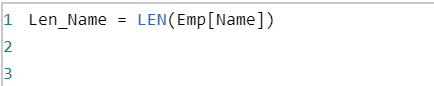Once you commit the DAX , a new column Len_Name is created  in EMP table.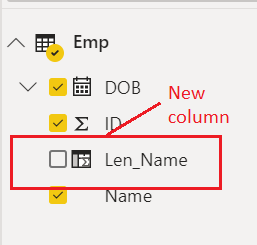Lets drag the Len_Name column into table visual, As you can see it counts the length of Name values.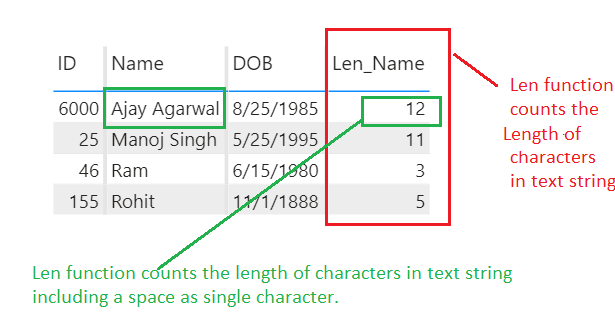## Len Function with integer data type

Lets see what is returned by Length function when it is used to calculate the length of an Integer values.

Here we have created a new column Len_Id in EMP table that uses a LEN function to calculate the length of Id.

`Len_ID = LEN(Emp[ID])`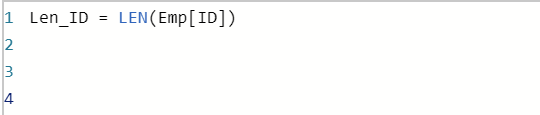Once you commit the DAX , a new column Len_Id  is created in EMP tableLets drag the Len_Id column into table visual to see the Length of Id, As you can see it does not count the actual length for an integer values provided by ID column.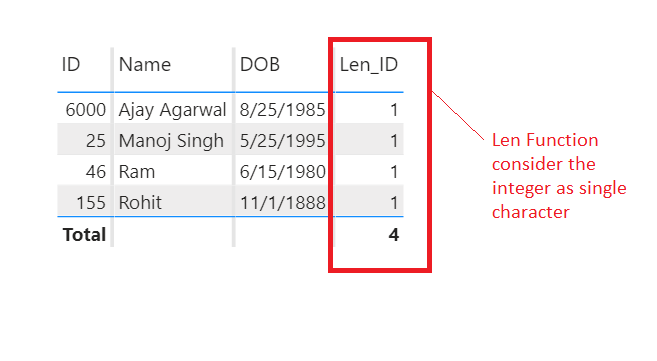## Len Function with Date data type

As Date type is not a text, so Len Function implicitly casts the value of date type to text using the current column format.

Lets create a new column Len_Date that uses the Len Function to calculate the Date values.

`Len_Date = LEN(Emp[DOB])`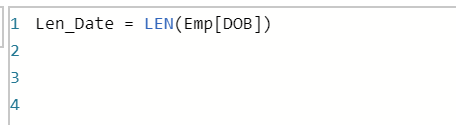Once you commit the DAX, it creates a new column in EMP table named as Len_Date.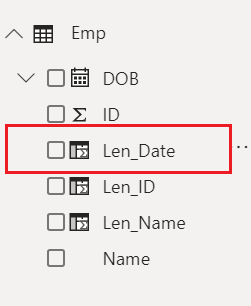Lets drag the Len_Date column into table visual, As you can see it returns the length of date values by implicitly casts the value of date type to text using the current column format.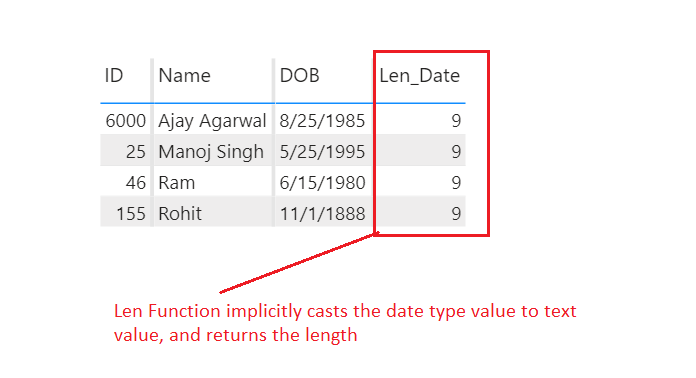This site uses Akismet to reduce spam. Learn how your comment data is processed.